1.子类和父类的相互赋值：

2.同名的成员变量

3.同名成员函数

1.构造函数

2.析构函数

3.拷贝构造

4.赋值运算符重载

四：单继承和多继承

多继承：

## 一：什么是继承

``````class human {//定义了一个父类，名字叫human
public:
string name = "小明";//父类里面定义了一个string类型的和一个int类型
int age = 18;
};
class student:public human {//定义了一个以public方式继承父类的子类student
public:
int schoolnum = 666;//在父类的name和age的基础上增加了一个schoolnum
void print()
{
cout << name << endl << age << endl << schoolnum << endl;//输出
}
};
int main()
{
student st;
st.print();
return 0;
}``````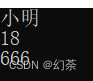好吧是不是还是看不懂，那让我们把这个代码分成两半

``````class human {
public:
string name = "小明";
int age = 18;
};``````

``````class student:public human {
public:
int schoolnum = 666;
void print()
{
cout << name << endl << age << endl << schoolnum << endl;
}
};``````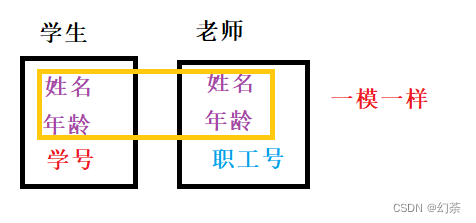所以为了偷懒提高效率，我们这里就可以把姓名和年龄封装到一个class类里面，也就是我们第一部分的代码，然后再新创一个类，继承原有姓名和年龄类的基础上，再新增学号/职工号，也就是我们第二类。

## 二：继承的格式

``class 新类的名字:继承方式 继承类的名字{};``

``````class student:public human{}；
//student是新类的名字，public是继承方式，human是要继承的类
//意思就是说我定义了一个名叫 student的类 以public的方式 来继承你human``````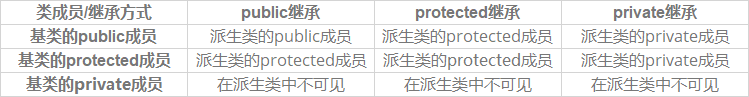我这里分享一个很巧妙的方式

``public>protectd>private``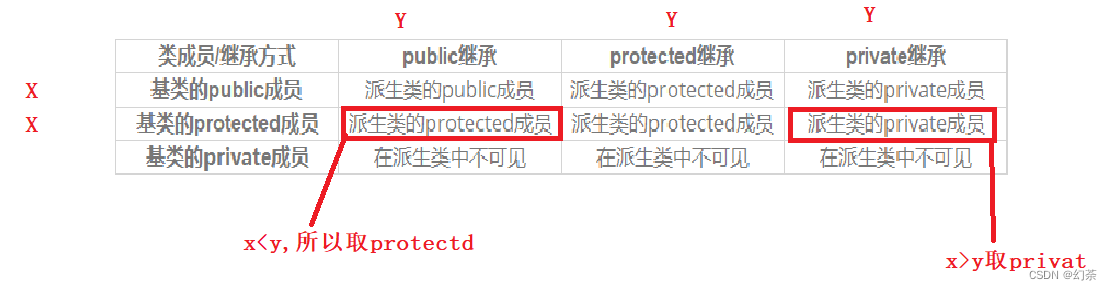最后一个private就都不可。这样我们就很轻松的记忆了下来

## 继承的总结：

1.基类private成员无论以什么方式继承到派生类中都是不可见的。这里的不可见是指基类的私有成员还是被继承到了派生类对象中，但是语法上限制派生类对象不管在类里面还是类外面都不能去访问它。
2.基类private成员在派生类中不能被访问，如果基类成员不想在派生类外直接被访问，但需要在派生类中访问，就定义为protected。可以看出保护成员限定符是因继承才出现的。
3.基类的私有成员在子类都是不可见；基类的其他成员在子类的访问方式就是访问限定符和继承方式中权限更小的那个（权限排序：public>protected>private）。
4.使用关键字class时默认的继承方式是private，使用struct时默认的继承方式是public，但最好显式地写出继承方式。

## 二：子类和父类（基类和派生类）

### 1.子类和父类的相互赋值：

``````class human {//父类
public:
string name = "小明";
int age = 18;
};
class student:public human {//子类
public:
int schoolnum = 666;
};
int main()
{
student st;
human hm;
hm = st;//将子类赋值给父类
st = hm;//将父类赋值给子类
return 0;
}``````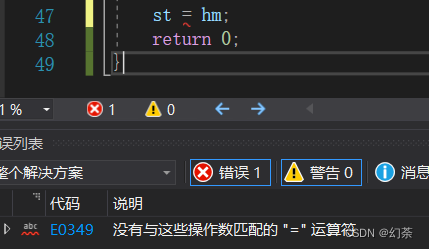在这里我们引入一个叫做切片原则的东西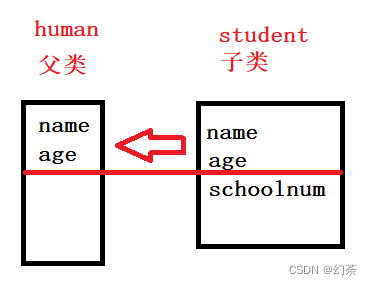因为父类中没有schoolnum，所以父类接收子类传过来的name和age之后，多余的schoolnum就不管了。但是如果父类传给子类，少传一个，所以会报错。

``````student st;//子类
human hm;//父类
hm = st;````````````student st;//子类
human& hm=st;父类``````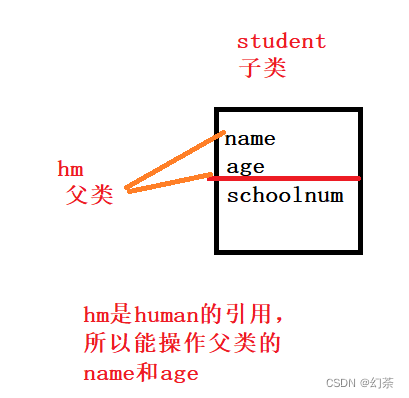``````student st;//子类
human* hm=&st;//父类``````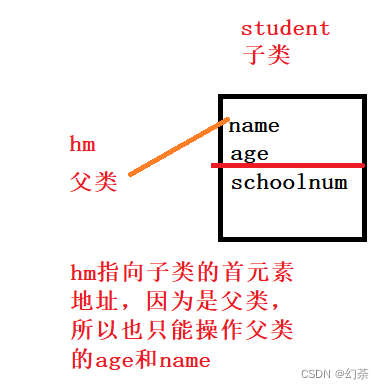### 2.同名的成员变量

``````class human {
public:
string name = "小明";
};
class student:public human {
public:
string name = "小红";
void print()
{
cout << name << endl;
}
};
int main()
{
student st;
st.print();
return 0;
}``````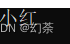``````void print()
{
cout << human::name << endl;
}``````

其实也很好理解，默认子类，父类就修饰限定

### 3.同名成员函数

``````class human {
public:
string name = "小明";
void print()
{
cout << name << endl;
}
};
class student :public human {
public:
string name = "小红";
void print()
{
cout << name << endl;
}
};
int main()
{
student st;
st.print();
return 0;
}``````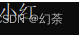但是我们修改一下子类的函数

``````void print(int x)//我们对子类的print函数加入一个参数
{
cout << name << endl;
}``````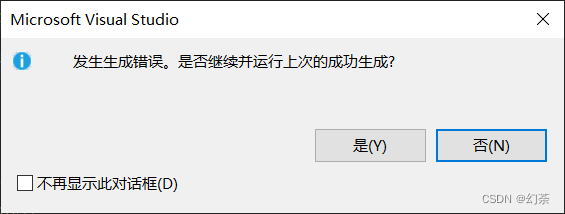这是因为编译器默认调用子类中print函数，但是子类中唯一的print函数有一个默认的参数，所以编译器无法找到匹配的print函数，所以就会报错。

## 三：子类中默认的成员函数

### 1.构造函数

``````class human {
public:
human(string name = "小明")//先调用：父类默认构造调用一个print打印name
:_name(name)
{
cout << name << endl;
}
protected:
string _name;
};

class student :public human {//后调用：子类默认构造调用一个print打印name和age
public:
student(string name,int age)
:_age(age)
{
cout << name << endl<<age<<endl;
}
protected:
int _age;
};

int main()
{
student st("小红", 18);
return 0;
}``````

结果如下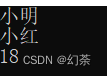可以看到，编译器先调用了父类的，打印出了小明，然后再次调用了子类的打印出了小红和age。

``````human(string name)//你这里不传值，那么就不能完成初始化，相当于父类失效
:_name(name)
{
cout << name << endl;
}``````

``````student(string name,int age)
:_age(age)
, human(name)//新增，子类以自己的name给父类的析构中的name赋值，age和name的顺序随意变动``````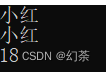实在不行就把父类的构造删了，反正编译器也默认会生成的

### 2.析构函数

``````class human {
public:
human(string name = "小明")
:_name(name)
{}
~human()
{
cout << "我是父类" << endl;
}
protected:
string _name;
};
class student :public human {
public:
student(string name,int a = 20)
:age(a)
{}
~student()

{
cout <<"我是子类"<< endl;
}
protected:
int age;
};
int main()
{
student st("小明", 18);
return 0;
}``````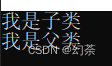所以说

### 3.拷贝构造

``````class human {
public:
human(string name="小明")
:_name(name)
{
cout << name << endl;
}
protected:
string _name;
};
class student:public human {
public:
student(string name, int age)
:_age(age)
{
cout << name << endl << age << endl;
}
student(student& s)
:human(s)//直接将st传过来通过切片拿到父类中的值
,_age(s._age)//拿除了父类之外的值
{
cout << s._age << endl<<s._name<<endl;
}
protected:
int _age;
};
int main()
{
student st("小红",18);
student st2(st);
return 0;
}
``````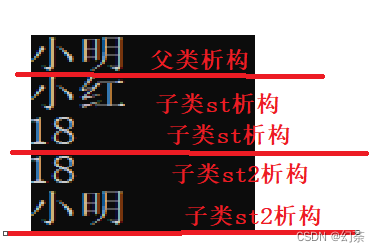### 4.赋值运算符重载

``````class human {
public:
human(string name = "小明")
:_name(name)
{
}
human& operator=(const human& p)
{
if (this != &p)
{
cout << "调用父类" << endl;
_name = p._name;
}
return *this;
}
protected:
string _name;
};
class student :public human {
public:
student(string name, int age)
:_age(age)
{
}
student(student& s)
:human(s)
, _age(s._age)
{
}
student& operator=(const student& s)
{
if (this != &s)
{
cout << "调用了子类" << endl;
human::operator=(s);//必须调用父类运算符
_age = s._age;
_name = s._name;
}
return *this;
}
protected:
int _age;
};
int main()
{
student st("小红", 18);
student st2(st);
student st3("小刚", 16);
st = st3;
return 0;
}
``````

结果如下：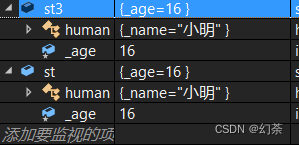## 四：单继承和多继承

### 单继承：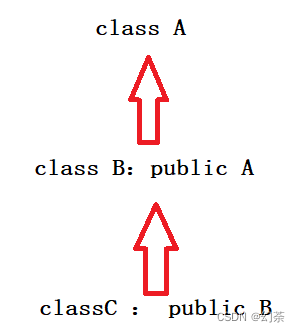### 多继承：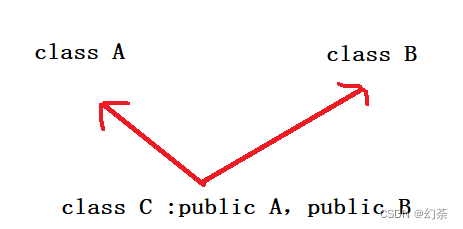由以上两点，我们就会发现一个很蛋疼厉害的继承，

### 菱形继承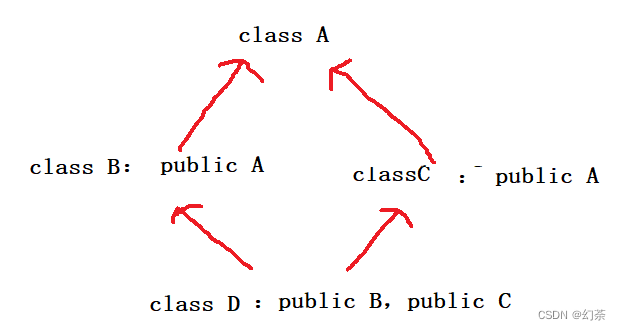``````class A {
public:
string name;
};
class B :public A {
public:
int age;
};
class C :public A {
public:
string sex;
};
class D :public B, public C {
public:
int id;
};
int main()
{
D student;
student.name = "小明";
student.age = 18;
student.sex = "男";
student.id = 666;
return 0;
}``````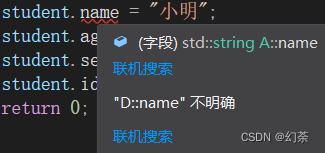因为这里的name，同时存在B和C中，所以D不知道继承B的name还是C中的name

### 解决方法一：

``student.B::name = "小明";``

### 解决方法二：

``````class B :virtual  public A {
public:
int age;
};
class C :virtual public A {
public:
string sex;
};``````

### 单继承和多继承的总结：